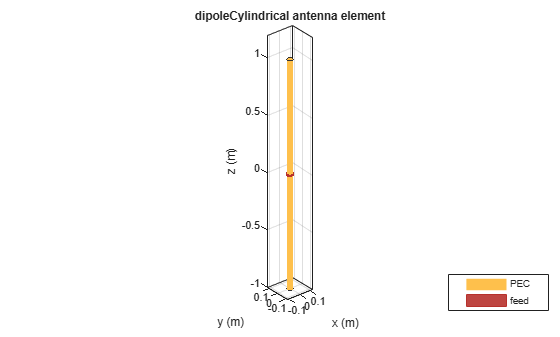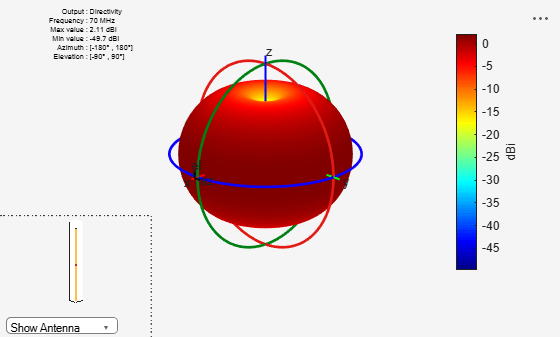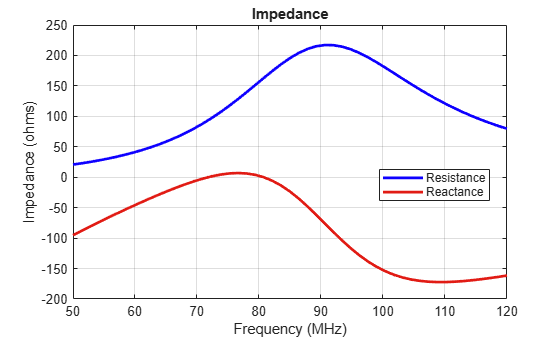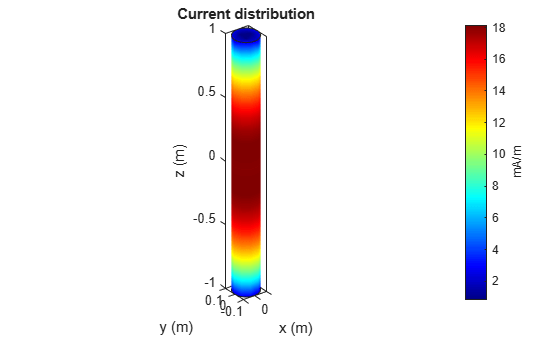# dipoleCylindrical

Create cylindrical dipole antenna

## Description

The `dipoleCylindrical` object creates a cylindrical dipole antenna. The length of the cylindrical dipole corresponds to half of the wavelength at the operating frequency. These antennas are used in designing thicker dipole antennas. These antennas are mostly used in wireless communications due to their simple design.## Creation

### Syntax

``ant = dipoleCylindrical``
``ant = dipoleCylindrical(Name,Value)``

### Description

example

``` `ant = dipoleCylindrical` creates a cylindrical dipole antenna object with default dimensions for an operating frequency of 70 MHz. The default dipole is center fed, with the feedpoint at the origin located on the y-z plane.```

example

````ant = dipoleCylindrical(Name,Value)` sets Properties using one or more name-value pairs. For example, `ant = dipoleCylindrical('Radius',0.04)` creates a cylindrical dipole antenna with radius of 0.04 meters.```

## Properties

expand all

Length of the dipole, specified as a positive scalar in meters.

Example: `'Length',3`

Data Types: `double`

Radius of the dipole, specified as a positive scalar in meters.

Example: `'Radius',0.05`

Data Types: `double`

Signed distance from the center along Z-axis, specified as a real-valued scalar in meters.

Example: `'FeedOffset',-0.2`

Data Types: `double`

Determine if the dipole ends are closed with a flat cap or left open, specified as `0` or `1`. Specify `1` for closed ends of the dipole and `0` for open ends.

Example: `'ClosedEnd',1`

Data Types: `double`

Type of the metal used as a conductor, specified as a metal material object. You can choose any metal from the `MetalCatalog` or specify a metal of your choice. For more information, see `metal`. For more information on metal conductor meshing, see Meshing.

Example: `m = metal('Copper'); 'Conductor',m`

Example: `m = metal('Copper'); ant.Conductor = m`

Tilt angle of the antenna, specified as a scalar or vector with each element unit in degrees. For more information, see Rotate Antennas and Arrays.

Example: `'Tilt',90`

Example: `ant.Tilt = 90`

Example: `'Tilt',[90 90]`,```'TiltAxis',[0 1 0;0 1 1]``` tilts the antenna at 90 degrees about the two axes defined by the vectors.

Data Types: `double`

Tilt axis of the antenna, specified as:

• Three-element vector of Cartesian coordinates in meters. In this case, each coordinate in the vector starts at the origin and lies along the specified points on the X-, Y-, and Z-axes.

• Two points in space, each specified as three-element vectors of Cartesian coordinates. In this case, the antenna rotates around the line joining the two points in space.

• A string input describing simple rotations around one of the principal axes, 'X', 'Y', or 'Z'.

Example: `'TiltAxis',[0 1 0]`

Example: `'TiltAxis',[0 0 0;0 1 0]`

Example: `ant.TiltAxis = 'Z'`

Data Types: `double`

Lumped elements added to the antenna feed, specified as a `lumpedElement` object handle. You can add a load anywhere on the surface of the antenna. By default, the load is at the feed. For more information, see `lumpedElement`.

Example: `'Load',lumpedelement`, where `lumpedelement` is the load added to the antenna feed.

Example: `ant.Load = lumpedElement('Impedance',75)`

## Object Functions

 `show` Display antenna or array structure; display shape as filled patch `axialRatio` Axial ratio of antenna `beamwidth` Beamwidth of antenna `charge` Charge distribution on metal or dielectric antenna or array surface `current` Current distribution on metal or dielectric antenna or array surface `cylinder2strip` Cylinder equivalent width approximation `design` Design prototype antenna or arrays for resonance around specified frequency `efficiency` Radiation efficiency of antenna `EHfields` Electric and magnetic fields of antennas; Embedded electric and magnetic fields of antenna element in arrays `impedance` Input impedance of antenna; scan impedance of array `mesh` Mesh properties of metal or dielectric antenna or array structure `meshconfig` Change mesh mode of antenna structure `optimize` Optimize antenna or array using SADEA optimizer `pattern` Radiation pattern and phase of antenna or array; Embedded pattern of antenna element in array `patternAzimuth` Azimuth pattern of antenna or array `patternElevation` Elevation pattern of antenna or array `rcs` Calculate and plot radar cross section (RCS) of platform, antenna, or array `returnLoss` Return loss of antenna; scan return loss of array `sparameters` Calculate S-parameter for antenna and antenna array objects `strip2cylinder` Calculates equivalent radius approximation for strip `vswr` Voltage standing wave ratio of antenna

## Examples

collapse all

Create a cylindrical dipole antenna with default properties.

`ant = dipoleCylindrical`
```ant = dipoleCylindrical with properties: Length: 2 Radius: 0.0250 FeedOffset: 0 ClosedEnd: 0 Conductor: [1x1 metal] Tilt: 0 TiltAxis: [1 0 0] Load: [1x1 lumpedElement] ```

View the antenna using the `show` function.

`show(ant)`Plot the radiation pattern of the cylindrical dipole antenna at a frequency of 70 MHz.

`pattern(ant,70e6)`Create a center-fed cylindrical dipole with a length of `2` m and a radius of `0.06` m.

`ant = dipoleCylindrical('Length',2,'Radius',0.06)`
```ant = dipoleCylindrical with properties: Length: 2 Radius: 0.0600 FeedOffset: 0 ClosedEnd: 0 Conductor: [1x1 metal] Tilt: 0 TiltAxis: [1 0 0] Load: [1x1 lumpedElement] ```

Plot the impedance over a frequency range of 50 MHz to 120 MHz.

`impedance(ant,linspace(50e6,120e6,51))`Create a cylindrical dipole antenna with a open-ended top and closed-ended top respectively.

```ant = dipoleCylindrical("Radius",0.1); ant_ClosedEnded = dipoleCylindrical("Radius",0.1,"ClosedEnd",1);```

Calculate and plot the current distribution for a cylindrical dipole antennas at 70MHz frequency.

`I_OpenEnded = current(ant,70e6)`
```I_OpenEnded = 3×400 complex -0.0000 - 0.0002i -0.0000 - 0.0005i -0.0000 - 0.0007i -0.0000 - 0.0005i -0.0000 - 0.0002i 0.0000 + 0.0002i 0.0000 + 0.0005i 0.0000 + 0.0007i 0.0000 + 0.0005i 0.0000 + 0.0002i 0.0000 + 0.0003i 0.0000 + 0.0007i 0.0000 + 0.0009i 0.0000 + 0.0007i 0.0000 + 0.0003i -0.0000 - 0.0003i -0.0000 - 0.0007i -0.0000 - 0.0009i -0.0000 - 0.0007i -0.0000 - 0.0003i -0.0000 - 0.0001i -0.0000 - 0.0002i -0.0000 - 0.0002i -0.0000 - 0.0002i -0.0000 - 0.0001i 0.0000 + 0.0001i 0.0000 + 0.0002i 0.0000 + 0.0002i 0.0000 + 0.0002i 0.0000 + 0.0001i 0.0000 + 0.0000i 0.0000 + 0.0001i 0.0001 + 0.0001i 0.0000 + 0.0001i 0.0000 + 0.0000i -0.0000 - 0.0000i -0.0000 - 0.0001i -0.0001 - 0.0001i -0.0000 - 0.0001i -0.0000 - 0.0000i -0.0000 - 0.0000i -0.0001 - 0.0001i -0.0001 - 0.0001i -0.0001 - 0.0001i -0.0000 - 0.0000i 0.0000 + 0.0000i 0.0001 + 0.0001i 0.0001 + 0.0001i 0.0001 + 0.0001i 0.0000 + 0.0000i 0.0000 + 0.0006i 0.0000 + 0.0004i 0.0000 + 0.0000i -0.0000 - 0.0004i -0.0000 - 0.0006i -0.0000 - 0.0006i -0.0000 - 0.0004i 0.0000 + 0.0000i 0.0000 + 0.0004i 0.0000 + 0.0006i -0.0000 - 0.0008i -0.0000 - 0.0005i 0.0000 + 0.0000i 0.0000 + 0.0005i 0.0000 + 0.0008i 0.0000 + 0.0008i 0.0000 + 0.0005i 0.0000 + 0.0000i -0.0000 - 0.0005i -0.0000 - 0.0008i 0.0000 + 0.0002i 0.0000 + 0.0001i 0.0000 + 0.0000i -0.0000 - 0.0001i -0.0000 - 0.0002i -0.0000 - 0.0002i -0.0000 - 0.0001i 0.0000 + 0.0000i 0.0000 + 0.0001i 0.0000 + 0.0002i -0.0001 - 0.0001i -0.0000 - 0.0001i 0.0000 + 0.0000i 0.0000 + 0.0001i 0.0001 + 0.0001i 0.0001 + 0.0001i 0.0000 + 0.0001i 0.0000 + 0.0000i -0.0000 - 0.0001i -0.0001 - 0.0001i 0.0001 + 0.0001i 0.0000 + 0.0000i 0.0000 + 0.0000i -0.0000 - 0.0000i -0.0001 - 0.0001i -0.0001 - 0.0001i -0.0000 - 0.0000i 0.0000 + 0.0000i 0.0000 + 0.0000i 0.0001 + 0.0001i -0.0191 - 0.0016i -0.0191 - 0.0016i -0.0191 - 0.0016i -0.0191 - 0.0016i -0.0191 - 0.0016i -0.0191 - 0.0016i -0.0191 - 0.0016i -0.0191 - 0.0016i -0.0191 - 0.0016i -0.0191 - 0.0016i -0.0190 + 0.0033i -0.0190 + 0.0033i -0.0190 + 0.0033i -0.0190 + 0.0033i -0.0190 + 0.0033i -0.0190 + 0.0033i -0.0190 + 0.0033i -0.0190 + 0.0033i -0.0190 + 0.0033i -0.0190 + 0.0033i -0.0189 + 0.0036i -0.0189 + 0.0036i -0.0189 + 0.0036i -0.0189 + 0.0036i -0.0189 + 0.0036i -0.0189 + 0.0036i -0.0189 + 0.0036i -0.0189 + 0.0036i -0.0189 + 0.0036i -0.0189 + 0.0036i -0.0185 + 0.0046i -0.0185 + 0.0046i -0.0185 + 0.0046i -0.0185 + 0.0046i -0.0185 + 0.0046i -0.0185 + 0.0046i -0.0185 + 0.0046i -0.0185 + 0.0046i -0.0185 + 0.0046i -0.0185 + 0.0046i -0.0183 + 0.0049i -0.0183 + 0.0049i -0.0183 + 0.0049i -0.0183 + 0.0049i -0.0183 + 0.0049i -0.0183 + 0.0049i -0.0183 + 0.0049i -0.0183 + 0.0049i -0.0183 + 0.0049i -0.0183 + 0.0049i ```
`current(ant,70e6)``I_ClosedEnded = current(ant_ClosedEnded,70e6)`
```I_ClosedEnded = 3×428 complex -0.0000 - 0.0002i -0.0000 - 0.0005i -0.0000 - 0.0007i -0.0000 - 0.0005i -0.0000 - 0.0002i 0.0000 + 0.0002i 0.0000 + 0.0005i 0.0000 + 0.0007i 0.0000 + 0.0005i 0.0000 + 0.0002i 0.0000 + 0.0003i 0.0000 + 0.0007i 0.0000 + 0.0008i 0.0000 + 0.0007i 0.0000 + 0.0003i -0.0000 - 0.0003i -0.0000 - 0.0007i -0.0000 - 0.0008i -0.0000 - 0.0007i -0.0000 - 0.0003i -0.0000 - 0.0001i -0.0000 - 0.0002i -0.0000 - 0.0002i -0.0000 - 0.0002i -0.0000 - 0.0001i 0.0000 + 0.0001i 0.0000 + 0.0002i 0.0000 + 0.0002i 0.0000 + 0.0002i 0.0000 + 0.0001i 0.0000 + 0.0000i 0.0000 + 0.0001i 0.0001 + 0.0001i 0.0000 + 0.0001i 0.0000 + 0.0000i -0.0000 - 0.0000i -0.0000 - 0.0001i -0.0001 - 0.0001i -0.0000 - 0.0001i -0.0000 - 0.0000i -0.0000 - 0.0000i -0.0001 - 0.0001i -0.0001 - 0.0001i -0.0001 - 0.0001i -0.0000 - 0.0000i 0.0000 + 0.0000i 0.0001 + 0.0001i 0.0001 + 0.0001i 0.0001 + 0.0001i 0.0000 + 0.0000i 0.0000 + 0.0006i 0.0000 + 0.0004i 0.0000 + 0.0000i -0.0000 - 0.0004i -0.0000 - 0.0006i -0.0000 - 0.0006i -0.0000 - 0.0004i 0.0000 + 0.0000i 0.0000 + 0.0004i 0.0000 + 0.0006i -0.0000 - 0.0008i -0.0000 - 0.0005i 0.0000 + 0.0000i 0.0000 + 0.0005i 0.0000 + 0.0008i 0.0000 + 0.0008i 0.0000 + 0.0005i 0.0000 + 0.0000i -0.0000 - 0.0005i -0.0000 - 0.0008i 0.0000 + 0.0002i 0.0000 + 0.0001i 0.0000 + 0.0000i -0.0000 - 0.0001i -0.0000 - 0.0002i -0.0000 - 0.0002i -0.0000 - 0.0001i 0.0000 + 0.0000i 0.0000 + 0.0001i 0.0000 + 0.0002i -0.0001 - 0.0001i -0.0000 - 0.0001i 0.0000 + 0.0000i 0.0000 + 0.0001i 0.0001 + 0.0001i 0.0001 + 0.0001i 0.0000 + 0.0001i 0.0000 + 0.0000i -0.0000 - 0.0001i -0.0001 - 0.0001i 0.0001 + 0.0001i 0.0000 + 0.0000i 0.0000 + 0.0000i -0.0000 - 0.0000i -0.0001 - 0.0001i -0.0001 - 0.0001i -0.0000 - 0.0000i 0.0000 + 0.0000i 0.0000 + 0.0000i 0.0001 + 0.0001i -0.0180 - 0.0011i -0.0180 - 0.0011i -0.0180 - 0.0011i -0.0180 - 0.0011i -0.0180 - 0.0011i -0.0180 - 0.0011i -0.0180 - 0.0011i -0.0180 - 0.0011i -0.0180 - 0.0011i -0.0180 - 0.0011i -0.0179 + 0.0038i -0.0179 + 0.0038i -0.0179 + 0.0038i -0.0179 + 0.0038i -0.0179 + 0.0038i -0.0179 + 0.0038i -0.0179 + 0.0038i -0.0179 + 0.0038i -0.0179 + 0.0038i -0.0179 + 0.0038i -0.0178 + 0.0041i -0.0178 + 0.0041i -0.0178 + 0.0041i -0.0178 + 0.0041i -0.0178 + 0.0041i -0.0178 + 0.0041i -0.0178 + 0.0041i -0.0178 + 0.0041i -0.0178 + 0.0041i -0.0178 + 0.0041i -0.0175 + 0.0052i -0.0175 + 0.0052i -0.0175 + 0.0052i -0.0175 + 0.0052i -0.0175 + 0.0052i -0.0175 + 0.0052i -0.0175 + 0.0052i -0.0175 + 0.0052i -0.0175 + 0.0052i -0.0175 + 0.0052i -0.0173 + 0.0055i -0.0173 + 0.0055i -0.0173 + 0.0055i -0.0173 + 0.0055i -0.0173 + 0.0055i -0.0173 + 0.0055i -0.0173 + 0.0055i -0.0173 + 0.0055i -0.0173 + 0.0055i -0.0173 + 0.0055i ```
```figure; current(ant_ClosedEnded,70e6)```